# Chapter 3: intoduction to statistics the Statistical Process

 Page 4/6 Date 03.05.2017 Size 341.52 Kb.

80 81 82 55 88 100

Even though the average for both groups is the same, the spread or variation of data in the Group B larger than Group A.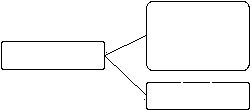(Syllabus focus on sample formula)

Sample variance and standard deviation

• For Ungrouped Data

Variance,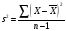Standard deviation,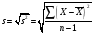where; X =individual value

=sample mean

n = sample size

OR

Variance,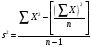Standard deviation,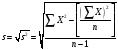(Note: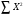is not the same as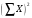)

Example:

1. The normal daily temperatures (in degrees Fahrenheit) in January for 10 selected cities are as follows. Find the variance and standard deviation.

50 37 29 54 30 61 47 38 34 61

1. Twelve students were given an arithmetic test and the times (in minutes) to complete it were

10 9 12 11 8 15 9 7 8 6 12 10

Find the variance and standard deviation.

• For Grouped Data

Variance,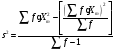Standard deviation,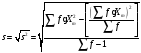(Students must show the working ie. Find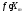and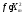)

Example:

1. In a class of 29 students, this distribution of quiz scores was recorded. Find variance and standard deviation.
 Grade Frequency 0 – 2 1 3 – 5 3 6 – 8 5 9 – 11 14 12 – 14 6

1. Eighty randomly selected light bulbs were tested to determine their lifetimes (in hours). This frequency distribution was obtained. Find variance and standard deviation.
 Class Boundaries Frequency 52.5 – 63.5 6 63.5 – 74.5 12 74.5 – 85.5 25 85.5 – 96.5 18 96.5 – 107.5 14 107.5 – 118.5 5

1. These data represent the scores (in words per minute) of 25 typists on a speed test. Find variance and standard deviation.
 Class limit Frequency 54 – 58 2 59 – 63 5 64 – 68 8 69 – 73 0 74 – 78 4 79 – 83 5 84 – 88 1

3.3.3 MEASURES OF POSITION

Standard scores, percentiles, deciles and quartiles are used to locate the relative position of the data value in the data set.

• Standard score / z-score

The z-score represent the number of standard deviations the data value is above or below the mean.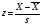Example:

1. Let data set : 65 , 70 , 75 ,80 , 85 ; =75 , s =5

65 70 75 80 85

-2s - s +2s +s

z= -2 z= -1 z= 0 z= 1 z= 2

For data value 83: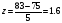1. Test marks are shown here. On which test she perform better?

Math marks: 65 50 45 ; =53.3 , s=10.4

Biology marks: 80 75 70 ; =75 , s=5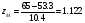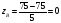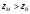, the relative position in math class is higher than her the relative position in biology class. She performs better in math paper than biology paper.

(the marks that she get from biology paper is more than mathematics paper but we cannot compare the marks directly because the papers are different i.e. number of question, standard of questions and so on, that is why we have to compare the relative position)